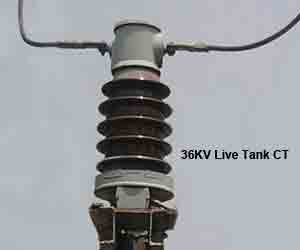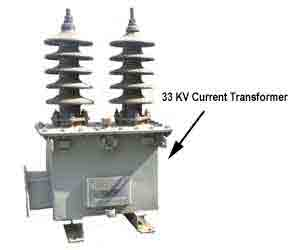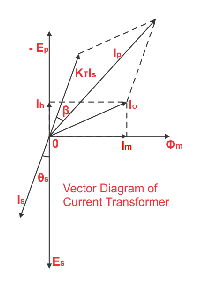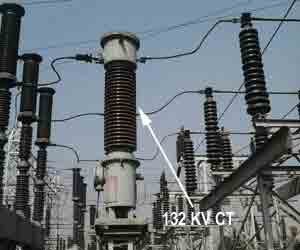# Current Transformer: What is it? (And How Does it Work?)

## Definition of Instrument Transformer

Instrument transformers means current transformer and voltage transformer are used in electrical power system for stepping down currents and voltages of the system for metering and protection purpose. Actually relays and meters used for protection and metering, are not designed for high currents and voltages.

High currents or voltages of electrical power system can not be directly fed to relays and meters. Current transformer steps down rated system current to 1 Amp or 5 Amp similarly voltage transformer steps down system voltages to 110 V. The relays and meters are generally designed for 1 Amp, 5 Amp and 110 V.

## What is a Current Transformer?

A current transformer (CT) is an instrument transformer in which the secondary current is substantially proportional to primary current and differs in phase from it by ideally zero degree.

## CT Accuracy Class or Current Transformer Class

A current transformer is similar to a electrical power transformer to some extent, but there are some difference in construction and operation principle. For metering and indication purpose, accuracy of ratio, between primary and secondary currents are essential within normal working range. Normally accuracy of current transformer required up to 125% of rated current; as because allowable system current must be below 125% of rated current.

Rather it is desirable the current transformer core to be saturated after this limit since the unnecessary electrical stresses due to system over current can be prevented from the metering instrument connected to the secondary of the current transformer as secondary current does not go above a desired limit even primary current of the current transformer rises to a very high value than its ratings. So accuracy within working range is main criteria of a current transformer used for metering purpose. The degree of accuracy of a metering current transformer is expressed by current transformer accuracy class or simply current transformer class or CT class.

But in the case of protection, the current transformer may not have the accuracy level as good as metering current transformer although it is desired not to be saturated during high fault current passes through primary.

So core of protection current transformer is so designed that it would not be saturated for long range of currents. If saturation of the core comes at lower level of primary current the proper reflection of primary current will not come to secondary, hence relays connected to the secondary may not function properly and protection system losses its reliability.Suppose, you have one current transformer with current ratio 400/1 A and its protection core is situated at 500 A. If the primary current of the current transformer becomes 1000 A the secondary current will still be 1.25 A as because the secondary current will not increase after 1.25 A because of saturation. If actuating current of the relay connected the secondary circuit of the CT is 1.5 A, it will not be operated at all even fault level of the power circuit is 1000 A.

The degree of accuracy of a protection current transformer may not be as fine as metering current transformer but it is also expressed by current transformer accuracy class or simply current transformer class or CT class as in the case of metering current transformer but in little bit different manner.## Theory of Current Transformer or CT

A CT functions with the same basic working principle of electrical power transformer, as we discussed earlier, but here is some difference. If a electrical power transformer or other general purpose transformer, primary current varies with load or secondary current. In case of CT, primary current is the system current and this primary current or system current transforms to the CT secondary, hence secondary current or burden current depends upon primary current of the current transformer.

In a power transformer, if load is disconnected, there will be only magnetizing current flows in the primary. The primary of the power transformer takes current from the source proportional to the load connected with secondary. But in case of CT, the primary is connected in series with power line. So current through its primary is nothing but the current flows through that power line.

The primary current of the CT, hence does not depend upon whether the load or burden is connected to the secondary or not or what is the impedance value of burden. Generally CT has very few turns in primary where as secondary turns is large in number. Say Np is number of turns in CT primary and Ip is the current through primary. Hence, the primary AT is equal to NpIp AT.

f number of turns in secondary and secondary current in that current transformer are Ns and Is respectively then Secondary AT is equal to NsIs AT.

In an ideal CT the primary AT is exactly is equal in magnitude to secondary AT.

So, from the above statement it is clear that if a CT has one turn in primary and 400 turns in secondary winding, if it has 400 A current in primary then it will have 1 A in secondary burden.

Thus the turn ratio of the CT is 400/1 A

## Current Transformer Error

But in an actual Current Transformer (CT), errors with which we are connected can best be considered through a study of phasor diagram for a CT,Is – Secondary current.
Es – Secondary induced emf.
Ip – Primary current.
Ep – Primary induced emf.
KT – Turns ratio = Numbers of secondary turns/number of primary turns.
I0 – Excitation current.
Im – Magnetizing component of I0.
Iw – Core loss component of I0.
Φm – Main flux.
Let us take flux as reference. EMF Es and Ep lags behind the flux by 90°. The magnitude of the passers Es and Ep are proportional to secondary and primary turns. The excitation current Io which is made up of two components Im and Iw.
The secondary current I0 lags behind the secondary induced emf Es by an angle Φ s. The secondary current is now transferred to the primary side by reversing Is and multiplied by the turns ratio KT. The total current flows through the primary Ip is then vector sum of KT Is and I0.

### The Current Error or Ratio Error in Current Transformer or CT

From above passer diagram it is clear that primary current Ip is not exactly equal to the secondary current multiplied by turns ratio, i.e. KTIs. This difference is due to the primary current is contributed by the core excitation current. The error in current transformer introduced due to this difference is called current error of CT or some times ratio error in current transformer.### Phase Error or Phase Angle Error in Current TransformerFor a ideal CT the angle between the primary and reversed secondary current vector is zero. But for an actual CT there is always a difference in phase between two due to the fact that primary current has to supply the component of the exiting current. The angle between the above two phases in termed as phase angle error in current transformer or CT.
Here in the pharos diagram it is β the phase angle error is usually expressed in minutes.

### Cause of Error in Current Transformer

The total primary current is not actually transformed in CT. One part of the primary current is consumed for core excitation and remaining is actually transformers with turns ratio of CT so there is error in current transformer means there are both ratio error in current transformer as well as a phase angle error in current transformer.

#### How to Reduce Error in Current Transformer

It is desirable to reduce these errors, for better performance. For achieving minimum error in current transformer, one can follow the following,

1. Using a core of high permeability and low hysteresis loss magnetic materials.
2. Keeping the rated burden to the nearer value of the actual burden.
3. Ensuring minimum length of flux path and increasing cross-sectional area of the core, minimizing joint of the core.
4. Lowering the secondary internal impedance.

Want To Learn Faster? 🎓
Get electrical articles delivered to your inbox every week.
No credit card required—it’s 100% free.